﻿ Relationship between Currency Depreciation and Trade Balance in India- An Econometric Study

### Relationship between Currency Depreciation and Trade Balance in India- An Econometric Study

Kanchan DattaOPEN ACCESSPEER-REVIEWED

## Relationship between Currency Depreciation and Trade Balance in India- An Econometric Study

Associate Professor of Economics, University of North Bengal

### Abstract

The effective exchange rate is a measure of whether or not the currency is appreciating or depreciating against a basket of foreign currencies. In this paper an attempt has been taken to enquire the relationship between exchange rate and trade balance in India, here we use we use 36 currency trade based effective exchange rate both nominal and real. A decline in the value of effective exchange rate implies a depreciation of the home currency against the basket of currencies. The data are taken from statistical hand book of published by RBI. The variables in this study are Trade Balance, Nominal Effective Exchange Rate, Real Effective Exchange Rate. Trade balance is measured by taking the ratio of import and export then converted in to logarithmic form, so trade balance implies log import – log export. This study shows that increase of trade balance of our country is one of the important reasons for depreciating our currency. Since trade balance is the ratio of import and export hence increase in trade balance implies more of import and or less of export is one of the important reasons for depreciation of our currency over the time period of the study.

### At a glance: Figures

123
Prev Next

• Datta, Kanchan. "Relationship between Currency Depreciation and Trade Balance in India- An Econometric Study." Journal of Finance and Economics 2.3 (2014): 83-89.
• Datta, K. (2014). Relationship between Currency Depreciation and Trade Balance in India- An Econometric Study. Journal of Finance and Economics, 2(3), 83-89.
• Datta, Kanchan. "Relationship between Currency Depreciation and Trade Balance in India- An Econometric Study." Journal of Finance and Economics 2, no. 3 (2014): 83-89.

 Import into BibTeX Import into EndNote Import into RefMan Import into RefWorks

### 1. Introduction

The exchange rate that prevails at a given date is known as the nominal exchange rate. It is amount of one currency interms of one unit of another currency. Since most of the countries of the world do not conduct all their trade with a single foreign country, policy makers are not so much concerned with what is happening to their exchange rate against a single foreign currency but rather what is happening to it against a basket of foreign currencies with which the country trades. The effective exchange rate is a measure of whether or not the currency is appreciating or depreciating against a basket of foreign currencies. While effective exchange rate provides a reasonable measure of changes in a countries competitive position for periods of several months, it does not take into account of the effect of price movements. In order to get a better idea of changes in countries competitive we would need to compile a real effective exchange rate index. In this paper we use 36 currency trades based effective exchange rate both nominal and real. A decline in the value of effective exchange rate implies a depreciation of the home currency against the basket of currencies. In this paper an attempt has been taken to enquire the relationship between exchange rate and trade balance in India. The data are taken from statistical hand book of published by RBI and they are monthly in nature. The variables in this study are Trade Balance (TBal), Nominal Effective Exchange Rate (NEER), Real Effective Exchange Rate (REER). Trade balnce is measured by taking the ratio of import and export then converted in to logarithmic form, so trade balance implies log import – log export. An increase of this difference implies more of import or less of export or both which actually reflects a deterioration of trade balance, on the other hand a decline of this log difference implies either increase in export or decrease in import or both which actually reflects an improvement of trade balance. All the other variables are also converted into logarithmic form. The time period covers from April 2009 to September 2013. Augmented Dickey Fuller, Phillips-Perron Unit root test, Johansen Co integration technique, Vector Error Correction model, Granger Causality etc. technique are used in this study.

### 2. Methodology

In econometric analysis, when time series data are used, the preliminary statistical step is to test the stationarity of each individual series. Unit root tests provide information about stationarity of the data. Nonstationarity data would contain unit roots. The main objectives of unit root tests is to determine the degree of integration of each individual time series.Results derived from the regression models would produce’Spurious Results’ if we use the data without checking their Stationarity Properties.

We can examine the stationarity of the datasets through following methods.

2.1. Augmented Dickey-Fuller Unit Root Test

In order to test for the existence of unit roots, and to determine the degree of differencing necessary to induce stationarity, we have applied the Augmented Dickey –Fuller test.Dickey and Fuller (1976, 1979), Said and Dickey (1984), Phillips (1987), Phillips and Perron (1988), and others developed modified version of the Dickey-Fuller tests when the error term i.e єt is not white noise.these tests are called “Augmented” Dickey- Fuller test (ADF). The results of the Augmented Dickey- Fuller test (ADF) help to determine the form in which the data should be applied in any econometric analysis. The alternative forms are as follows.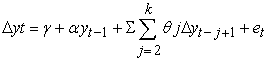(1)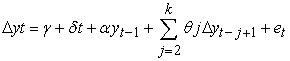(2)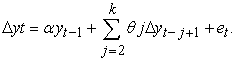(3)

where, y t = Model Variables Δ yt = First differenced series of y t.

Δ y t-j+1 = First differenced series of yt at (t-j+1) th lags. (j = 2----k)

The equation (1) contains a constant as exogenous, while equation (2) bears a constant along with a linear trend. However, equation (3) presents an auto- regressive process with no constant and linear trend.

2.2. Phillips –Perron Unit Root Test

Phillips (1987), Phillips and Perron (1988) have generalized the Dickey –Fuller (DF) tests to the situations where disturbance processes, є t are serially correlated. The PP is intended to add a ‘correction factor’ to the DF test statistic.

Let the AR (1) model be,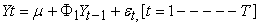(4)

With Var (є t) ≡ σ2 є

If є t is serially correlated, the ADF approach is to add lagged ΔY t to ‘whiten’ the residuals. To illustrate the alternative approach, the test statistic T (Ф1-1) has been considered which is distributed as ρμ in the maintained regression with an intercept but no time trend. The PP modified version is,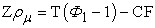(5)

where the correction factor CF is,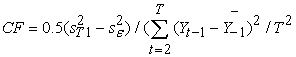(6)

and,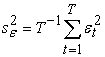(7)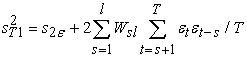(8)

where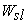= 1-s/(1+ l) and є t = Y t-μ -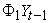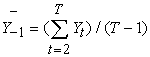(9)
2.3. Test of Co-integration

The co-integration test represents the gesticulation of long run equilibrium relationship between two variables say yt and xt let both are integrated at one, that is y t ~ I(1) and xt ~ I(1). Then yt and xt are said to be cointegrated if there exist a β such that y t - β xt is I (0). This is denoted by saying yt and xt are CI (1,1).that is yt and xt are cointegrated. Different types of co-integration techniques are available for the time series analysis. These tests include the Engle and Granger test (1987), Stock and Watson procedure (1988) and Johansen’s method (1988). This study uses Johansen technique of cointegration.

The Johansen maximum likelihood procedure analyses the relationship among stationary or non-stationary variables using the following equation: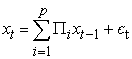(10)

The function can be presented according to the following VAR system: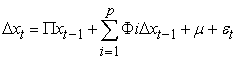(11)

In which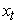is an n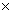1 random vector, є t is NIID (0,σ2є), and μ is deterministic terms. The long run relationships are derived through the coefficient Matrix of П, denoted by r, which is between 0 and n. Then there are r linear combinations of the variables in the system that are I(0) or co-integrated. Under Johansen (1991), and Johansen and Juselius (1990) procedures, two tests are available for the determination of co-integrating vectors and for the estimation of their values. These tests are the Trace Test and the Eigen Value Test. In this method, a two-stage testing procedure has been followed. In the first stage, the null hypothesis of no Cointegration hypothesis is tested against the alternative hypothesis that the data are cointegrated with an unknown cointegrating vector. If the null hypothesis is rejected, a second stage test is implemented with Cointegration maintained under both the null and alternative. Gonzalo (1994) has suggested that Johansen’s procedure has certain properties which are superior to alternative co-integrating testing methods.

### 3. Empirical Findings

Time plots of NEER (Figure1) shows that in April 2009 exchange rate increases for few time and then declines at least for three months, then again from the middle of 2009 it increases and this increase stays for another 4 to 5 months, from the end of 2009 it starts to decline and it continues to decline through out the time horizon of the study except for few periods. Figure-2 shows the time plots of real effective exchange rate which shows an increase up to middle of 2010 starting from the study period April 2009 but after that there is a continuous fall through out the study periods except for one or two periods increase. Since the rate of change of NEER or REER implies the magnitude of depreciation or appreciation figure-3 shows the time plots of 1st difference of log nominal effective and log real effective exchange rate which is nothing but the rate of change of exchange rate that is appreciation or depreciation. Figure-4 shows the time plots of Trade Balance which clearly shows a downward pattern upto October 2010, with certain ups and down in between but after that that is from the end of 2010 to the end of the study period there is a clear upward movement. Since upward movement implies more of import compared to export so it means more trade deficit.

The correlogram structure (Figure 5 to Figure 8) of the variables show that both the REER and NEER are non-sationary at level but they become stationary after 1st difference, but Figure-9 shows that the correlogram structure of trade balance is stationary at level. Correlogram structure provides a rule of thumb idea about the stationarity or non stationarity of the time series variables, but for more perfection we generally depend on Augmented Dickey-Fuller Test (ADF Test) and Phillips-Perron Unit root test (the results of ADF and PP unit root test are shown in Table 1).

#### Table 1. ADF and PP Unit Root Test on TBAL, NEER and REER

In both the ADF and PP test it is clear that Trade Balance is stationary at level but both the exchange rate Nominal effective exchange rate and the Real effective exchange Rate are I(1) variable that is they are non stationary at level but becomes stationary after 1st difference. Since 1st difference of log is growth hence we are taking growth of NEER ad or growth of REER as one variable and Trade Balance at level is another variable of this study. Since graphical representation of both the exchange rate shows a declining trend (if there is continuous depreciation) hence its rate of change implies depreciation. So trade balance (TRADEBAL) and growth of nominal or real effective exchange rate that is DREER and or DNEER as depreciation of exchange rate (DEPEXR) as another variable in this study, hence both the variables becomes same order of integration. Hence these two variables are automatically cointegrated. But still for confirmation we run Johansen Cointegration Test (the results are shown in the Table 2) which clearly shows that there exist cointegrating relation between DNEER and TRADEBAL. Therefore there exist a long-run relationship between depreciation of nominal or real effective exchange rate and trade balance in the economy of India for the time horizon of the study.

#### Table 3. Results of Johansen’s cointegrating between Depreciation and Trade Balance (Series DLREER TRADEBAL)

Cointegration implies long-run relationship between the variables but in the short-run there may be deviations, hence the stability of the long-run relationship can be expressed by estimating vector error correction model (VECM). This model combines the short term effect as well as long-run relation by incorporating one error correction term (one period lagged error series of the cointegrating relation). The vector error correction model can be written as follows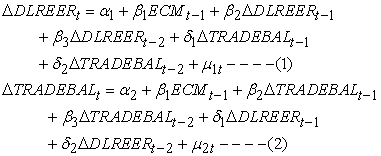The estimated coefficient of the error correction term implies the speed of adjustment if there is any significant deviation from long-run equilibrium relationship. The results of the VECM are shown in the Table 4 below.

#### Table 4. results of the Vector Error Correction Model

From the above results it is clear that one of the ECM coefficients is negative and less than one and it is statistically significant. It implies the stability of the long –run relationship. The speed of adjustment is 0.54, it implies 54% deviations of the previous period is autometically corrected in the present period. More over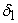and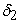in the 1st equation is negative and statistically significant. It implies increase of trade balance (both in last one and two periods) tries to reduce the growth of real effective exchange rate, since reduction of REER means more deprecation, hence it shows that increase of trade balance of our currency is one of the reasons for depreciating our currency. Since trade balance is the ratio of import and export hence increase in trade balance implies more of import and or less of export is one of the important reasons for depreciation of our currency at least in the short run over the time period of the study. VEC Pairwise Granger Causality Test that is based on chi-square statistic also supports that in the short run Trade balance Granger Causes depreciation. This is shown in the Table 5. On the other hand since depreciation is not significantly affecting trade balance hence the Marshall-Lerner Condition is not satisfied in India

### 4. Diagnostic Checking

One of the important criteria for testing the goodness of the estimated VEC model is the normality of residual of the estimated regression. This is done through Jarque-Berra Statistic. The table below shows the results of the normality test which clearly shows the acceptance of the null hypothesis. Hence the residuals of the estimated VEC model is normal (JB statistic is 4.94 with high probability value). Similarly the tests for autocorrelation of the error series are shown by Portmanteau Tests (Table 7). The test is valid only for lags larger than the VAR lag order. This test also shows the acceptance of the null hypothesis that is no residual autocorrelations up to lag 12. The results are almost same if we take nominal effective exchange rate instead of real effective exchange rate with a little bit difference in magnitude. All these show that our estimation is reliable.

### 5. Summary and Conclusion

The effective exchange rate is a measure of whether or not the currency is appreciating or depreciating against a basket of foreign currencies. While effective exchange rate provides a reasonable measure of changes in a countries competitive position for periods of several months, it does not take into account of the effect of price movements. In order to get a better idea of changes in countries competitive we would need to compile a real effective exchange rate index.

In this paper we use 36 currency trades based effective exchange rate both nominal and real. A decline in the value of effective exchange rate implies a depreciation of the home currency against the basket of currencies. This paper finds that increase of trade balance (both in last one and two periods) tries to reduce the growth of real effective exchange rate, since reduction of REER means more deprecation, hence it shows that increase of trade balance of our currency is one of the reasons for depreciating our currency. Since trade balance is the ratio of import and export hence increase in trade balance implies more of import and or less of export is one of the important reasons for depreciation of our currency at least in the short run over the time period of the study. So to strengthen our currency first of all we have to think we are Indian, our domestic product is much better than imported one. The most important thing is honesty. Unless corruptions are curved from the apex level, negative trade balance can never be reversed in India.

### References

  Bahmani-Oskooee M. 1991. Is there a long-run relationship between the trade balance and the real effective exchange rate o LDCs? Economics Letters, 36:403-407.In article CrossRef  Bahmani-Oskooee, Mohsen, Devaluation and the J-Curve: Some evidence from LDCs, 1985, The Review of Economics and Statistics, Vol. 67, August, 500-504.In article  Bahmani-Oskooee, Mohsen, 1986, Determinants of international trade flows: The case of developing countries, Journal of Development Economics 20, 107-123.In article CrossRef  Bahmani-Oskooee, M. and M. Malixi, 1987, Effects of exchange rate flexibility on the demand for international reserves, Economics Letters 23, 89-93.In article CrossRef  Bahmani-Oskooee, M. and Gour Gobinda Goswami. 2004. Exchange rate sensitivity of Japan’s bilateral trade flows,” Japan and the World Economy, 16: 1-15.In article CrossRef  Baharumshah, A. Zubaidi. 2001. The Effect of Exchange Rate on Bilateral Trade Balance: New Evidence from Malaysia and Thailand. Asian Economic Journal, 15, (3):291-312.In article CrossRef  Buluswar, Murli D., Henry T. and K. P. Upadhaya. 1996. Devaluation and the trade balance in India: stationarity and cointegration. Applied Economics, 28: 429-432.In article CrossRef  Dickey, David A., William R. Bell and Robert B. Miller, 1986, Unit roots in time series models: Tests and implications, The American Statistician 40, 12-26.In article  Engle, Robert F. and C.W.J. Granger, 1987, Co-integration and error correction: Representation, estimation, and testing, Econometrica 55, 251-276.In article CrossRef  Fuller, Wayne A., 1976, Introduction to statistical time series (Wiley, New York).In article  Granger, C.W.J., 1986, Developments in the study of cointegrated economic variables, Oxford Bulletin of Economics and Statistics 48, 213-228.In article CrossRef  Haynes, Stephen E. and Joe A. Stone, 1982, Impact of the terms of trade on the U.S. trade balance: A reexamination, The Review of Economics and Statistics 64, 702-706.In article CrossRef  Himarios, Daniel, 1985, The effects of devaluation on the trade balance: A critical view and reexamination of Miles’ new results, Journal of International Money and Finance 4, 553-563.In article CrossRef  Himarios, Daniel, 1989, Do devaluations improve the trade balance? The evidence revisited, Economic Inquiry 27, 143-168.In article CrossRef  Houthakker, Hendrik S. and Stephen Magee, 1969, Income and price elasticities in world trade, Review of Economics and Statistics 51, 111-125.In article CrossRef  Khan, Mohsin, 1974, Import and export demand in developing countries, IMF Staff Papers 21, 678-693.In article CrossRef  Miles, Marc A., 1979, The effects of devaluation on the trade balance and the balance of payments: Some new results, Journal of Political Economy 87, 600-620.In article CrossRef  Warner, Dennis and Mordechai E. Kreinin, 1983, Determinants of international trade flows, Review of Economics and Statistics 65, 96-104.In article CrossRef